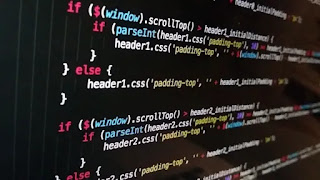# Python Return vs Print | Difference between return and print - codebugfree

In this section we discuss the python return vs print. Mainly beginner confused in python return and print function. Return and print is the built in function of python.

Return and print is a different is not a same. Print is use printing the variable , method, function. And Return is use to return the value to method or function then print that method or function.Python return vs print

### Where to use print and return

When you make a def function and using the return to return the value than when def function call  must use print.

As same of  upper case use def function in print. function call with out using print. ALs work same  in OOPs concept but when using the property method without usinig return or print no need to use print when call the function.

When programmer use the property method in OOPs concept they call function with out using print and return. Programmer only call the function.

If use return in def function than you only call the function than this case print the NONE  return does not give any value to def function.

Now we see the code to identify the difference between python return and print.

``````def return_vs_print():
return "learn python from code bug free"
print(return_vs_print())

def print_vs_return():
print("Learn the python")
print_vs_return()

def return_out_print():
print("Return nome when function call")
print(return_out_print())
``````

In first function return the value ti function and print. Second def function print the value and call the function. In last def function print the value and print the function in this case print the function call the return value. So, here is not a use return then function return the none.

INOUTPUT

``````learn python from code bug free
Learn the python
Return nome when function call
None``````

Now we see the code of  OOPs concept using print vs else

``````class _return_print():
def __init__(self,name,langauge):
self.name = name
self.language = langauge
def print_me(self):
print(f"my name is {self.name} and my langauage is {self.language}")

def return_me(self):
return f"my name is {self.name} and my language is {self.language}"

codebugfree = _return_print("vs code","python")#object
codebugfree.print_me()
print(codebugfree.return_me())``````

Here is use opps concept first make the class inside the class making the init constructor make def function to return and print the value. making object inside pass the init constructor attributes and print the function.

INOUTPUT

``````my name is vs code and my langauage is python
my name is vs code and my language is python``````